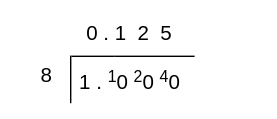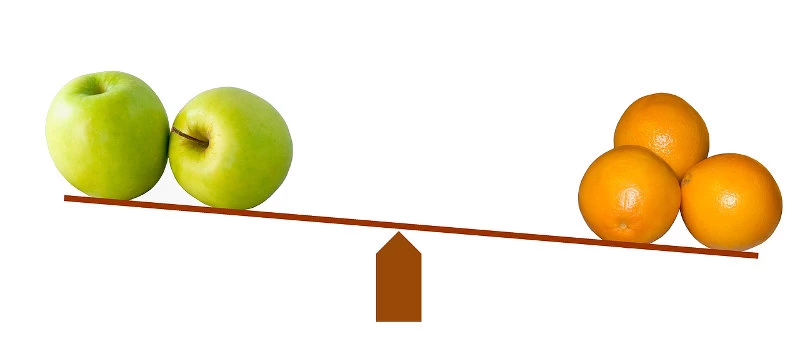# Fraction to Decimal Calculator

## Converting fractions to decimals is easier than you think!Converting simple fractions, like 1/4 or 1/2, to decimal forms is rather simple. However, tackling bulkier fractions can be quite a challenge.

Thankfully, the CalcoPolis fraction-to-decimal calculator is a nifty tool that can simplify the process for you. It can even help you round up your values!

If you wish to reverse this operation, you may use our decimal to fraction converter.

## How to Convert Fractions to Decimals?

To understand how to convert fractions to decimals, let’s look at 13/104 as an example.

First, you’ll need to reduce the fraction to its simplest form. That’s 1/8.

Next, you’ll go over a short division calculation to convert 1/8 to decimal form. If you’re not sure how to do that, you can refresh your memory quickly, but the process of dividing and carrying remainders should look like this:Of course, if you’ll use the fraction-to-decimal calculator, you won’t need to do any of that.

Instead, you just input “13” into the numerator slot and “104” into the denominator slot and choose a precision setting. In this case, the thousandth (3 decimals) will do just fine. Then, you can click “calculate” to complete the conversion and get the result “0.125” in a blink.

## Why Convert Fractions to Decimals?

Although the tool is fairly easy to use, you might be wondering when it’ll come in handy. On our other page, you can find the tool that lets you convert fractions to a percentage.

Here are some common reasons to convert fractions to decimals:

### Doing Simple Calculations

Adding and subtracting decimals is rather straightforward. You can do the calculations just like you would with whole numbers, but you’ll just need to keep track of where the decimal point goes.

Meanwhile, fractions can make some calculations complex.

Suppose you wanted to add 49/4 and 72/5. First, you’d need to get common denominators by turning the fractions into 245/20 and 288/20, respectfully. Then, you add them to get 533/20 or 26.65.

On the other hand, using decimals simplifies the process drastically because you no longer have to worry about common denominators. With a fraction-to-decimal converter, you’d just turn the 49/4 to 12.25 and the 72/5 to 14.4.

Now, 12.25 and 14.4 are much easier to add up, and you’ll get the same result of 26.65.

### Using Calculator Apps

There’s a wide range of online tools that can help you with complex equations. You just need to find a calculator tailored to your needs, input the values into empty slots, and press a button to get the results.

Unfortunately, not all of those calculators will allow you to input fractions. Instead, you might need to convert the values to decimals first to be able to type them into the tool’s interface.

### Comparing ValuesSure, sometimes fractions can be more accurate for mathematical calculations. After all, you won’t always be able to get terminating decimals after dividing every numerator and denominator.

Yet, decimals can make more sense for day-to-day purposes as long as you don’t really mind approximated values.

For instance, values like 443/45 and 89/9 can seem too bulky and far off from each other at first glance. However, once you turn them into decimals, you’ll see that their approximate values are actually 9.84 and 9.89.

Similarly, fractions like 2/5 and 3/8 can be a bit misleading when you’re trying to compare them. Instead, it can be easier to convert them to decimals to find out that they represent 0.4 and 0.375, respectively.

So, 2/5 is clearly larger than 3/8 when you see the values laid out as decimals. On the other hand, not everyone will be able to realize that just by looking at the fractions.

## Similar tools

If you wish to simply round fractions to the nearest integer you may use our rounding fractions calculator. Alternatively, if you deal with complex fractions, you can simplify them using our fraction simplifier, which will reduce the fraction or a mixed number using a common denominator.

Math can be tricky, and even simple calculations may lead to silly mistakes. You can use tools available in the math category (for example percent to fraction calculator) to double check if your calculations are correct.

### Authors

Created by Lucas Krysiak on 2022-11-21 16:53:53 | Last review by Mike Kozminsky on 2022-12-09 11:41:12